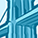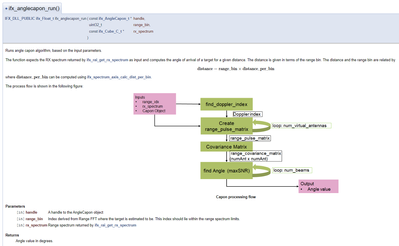Level 1Level 1

# Get angle capon information by calling ifx_anglecapon_run in AngleCapon.c

Hi, I am developing an application to extract angle capon information based on rx data read from antannas.
I know that there is no sample code for anglecapon, so I am writing C code to try out.

I modify from the sample code app_raw_data.c in the following steps.
1. fetch radar data by ifx_avian_get_next_frame(ifx_Avian_Device_t* device_handle, ifx_Cube_R_t* frame), the data stored in "frame".
2. call ifx_anglecapon_run(const ifx_AngleCapon_t* handle, uint32_t range_idx, ifx_Cube_C_t* rx_spectrum, uint32_t* angle_idx) to run angle capon algo.

My questions are,
1. to call function ifx_anglecapon_run, how to define range_idx and angle_idx?
2. should I copy from the parameter of "frame" fetched from 1 and copy to input parameter rx_spectrum?
3. input parameter rx_spectrum is decleared as ifx_Cube_C_t while "frame" is ifx_Cube_R_t, is there a way to transfer from ifx_Cube_R_t to ifx_Cube_C_t?

Cheng WC

1 SolutionModeratorModerator

# Re: Get angle capon information by calling ifx_anglecapon_run in AngleCapon.c

Hi @chengwc ,

The range index (range_idx) is the range FFT bin you estimate the target to be on and angle index (angle_idx) is the output of the function ifx_anglecapon_run. The range_idx needs to be computed as the result of a peak search over the absolute range values.

As for ifx_Cube_C_t* rx_spectrum, this needs to be populated by complex range FFT values as follows
number of slices --> number of rx antennas (2)
number of rows --> length of range_fft
number of columns --> number of chirps

Best regards,

Deepa

6 RepliesModeratorModerator

# Re: Get angle capon information by calling ifx_anglecapon_run in AngleCapon.c

Hi @chengwc ,

The range index (range_idx) is the range FFT bin you estimate the target to be on and angle index (angle_idx) is the output of the function ifx_anglecapon_run. The range_idx needs to be computed as the result of a peak search over the absolute range values.

As for ifx_Cube_C_t* rx_spectrum, this needs to be populated by complex range FFT values as follows
number of slices --> number of rx antennas (2)
number of rows --> length of range_fft
number of columns --> number of chirps

Best regards,

DeepaLevel 1Level 1

# Re: Get angle capon information by calling ifx_anglecapon_run in AngleCapon.c

Hi all,

Thanks for your replies and information.

I tried testing angle capon by modifying the application code app_presence_detection.c since it looks comprehensive.
What I did are shown below.

1. configure an angleCapon handler by calling config_angleCapon(void)
2. run the app_presence_detection as normal.
3. in the function presence_process, copy the corresponding data from the ps_result to rx_spectrum as following, then I call function ifx_anglecapon_run to get result.

My questions are:
1. My understanding is that function ifx_presence_sensing_run form a structure ps_result.range_spectrogram, which contains result data after fft. Is my understanding correction?

2. To use Anglecapon, can I do in Matlab other than C?

The testing code  are shown below.
ifx_AngleCapon_t* g_angleCapon_handle;

ifx_AngleCapon_t* config_angleCapon(void) {
ifx_AngleCapon_Config_t angleCapon_conf;
ifx_AngleCapon_t* angleCapon_handle;

//CHENG: definition as in Documentment Data Structure
angleCapon_conf.chirps_per_frame = 4;
angleCapon_conf.d_by_lambda = 1;
angleCapon_conf.max_angle_degrees = 90;
angleCapon_conf.min_angle_degrees = 90;
angleCapon_conf.num_beams = 1;
angleCapon_conf.num_virtual_antennas = 2;
angleCapon_conf.phase_offset_degrees = 90;
angleCapon_conf.range_win_size = 10;
angleCapon_conf.selected_rx = 2;

angleCapon_handle = ifx_anglecapon_create(&angleCapon_conf);
if (angleCapon_handle == NULL) {
printf("CHENG: angleCapon_handle return NULL...\n");
exit();
}
return angleCapon_handle;
}

ifx_Error_t presence_process(presence_t* presence_context, ifx_Cube_R_t* frame)
{
ifx_PresenceSensing_Result_t ps_result = { 0 };
ifx_Matrix_R_t antenna_data;

ifx_cube_get_row_r(frame, RX_ANTENNA_ID, &antenna_data);
ifx_presence_sensing_run(presence_context->presencesensing, &antenna_data, &ps_result);
if (ifx_error_get() != IFX_OK)
return ifx_error_get();

//CHENG: start
{
g_angleCapon_handle = config_angleCapon();
printf("CHENG: presence_process(), frame rows is %d\n", IFX_CUBE_ROWS(frame));
ifx_Cube_C_t rx_spectrum;
ifx_Float_t runResult;
uint32_t angle_idx;
uint32_t range_idx=100;

rx_spectrum.rows = ps_result.range_spectrogram->rows;
rx_spectrum.cols = ps_result.range_spectrogram->cols;
rx_spectrum.slices = frame->rows;
rx_spectrum.owns_d = ps_result.range_spectrogram->owns_d;
rx_spectrum.d = (ps_result.range_spectrogram->d);
ifx_anglecapon_run(&g_angleCapon_handle, range_idx, &rx_spectrum, &angle_idx);
}
//CHENG: endEmployeeEmployee

# Re: Get angle capon information by calling ifx_anglecapon_run in AngleCapon.c

Hi @chengwc

The Angle Capon algorithm expects the Capon object which was created using ifx_anglecapon_create() and the range_idx and rx_spectrum values extracted from the latest radar measurement.

• range_idx: Index derived from Range FFT where the target is estimated to be. This index should lie within the range spectrum limits.
• rx_spectrum: Range spectrum for all RX channels this is an ifx_Cube_C_t where (rows, columns, slices) correspond to (Range bins, Chirps, Rx Channels)
• angle_idx: Angle index that indicates the target from the number of configured beams.

Hope that helps.

Cheers,
Montassar.EmployeeEmployee

# Re: Get angle capon information by calling ifx_anglecapon_run in AngleCapon.c

Hello @chengwc,

we have improved the documentation for AngleCapon. The documentation also applies to the last released version with the only difference that angle_idx has been removed in the non-released version:I hope this helps,

--MichaelLevel 1Level 1

# Re: Get angle capon information by calling ifx_anglecapon_run in AngleCapon.c

Hi Michael,

I have just downloaded latest version 2022.6.1 from Infineon Developer Center Launcher (https://softwaretools.infineon.com/tools/com.ifx.tb.launcher2), and the function prototype for ifx_anglecapon_run still contains angle_idx, and I cannot find the diagram you shown in the documents.

May I know how I can get the version you mentioned?

ThanksEmployeeEmployee

# Re: Get angle capon information by calling ifx_anglecapon_run in AngleCapon.c